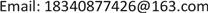1. 引言

2. 模型介绍2.1. 逻辑回归模型

P ( Y = 1 | x ) = π ( x ) ,     P ( Y = 0 | x ) = 1 − π ( x ) (1)

∏ i = 1 N [ π ( x i ) ] y i [ 1 − π ( x i ) ] ( 1 − y i ) (2)

L ( w ) = ∑ i = 1 N [ y i log π ( x i ) + ( 1 − y i ) log ( 1 − π ( x i ) ) ] = ∑ i = 1 N [ y i log π ( x i ) 1 − π ( x i ) + log ( 1 − π ( x i ) ) ] = ∑ i = 1 N [ y i ( w ∗ x i ) − log ( 1 + exp ( w ∗ x i ) ) ] (3)

P ( Y = 1 | x ) = e w ¯ x 1 + ( e w ¯ x ) (4)

P ( Y = 0 | x ) = 1 1 + ( e w ¯ x ) (5)

2.2. 随机森林模型

2.3. XGBoost模型

XGBoost模型是Chen等人在2016年提出的一种Boosting模型 ，在传统GBDT算法的基础上，对损失函数进行二阶泰勒展开，并且加入了正则化项，平衡模型的复杂度和的目标函数的下降速度，能够有效解决过拟合问题。Boosting的思想是将多个弱学习分类器集合起来形成一个强学习分类器，而XGBoost所用到的树模型是CART树。XGBoost通过不断的添加树来分裂特征，每添加一棵树就是在学习一个新函数来拟合上个函数的残差。在对数据进行预测时，就是这个数据的每个特征都会落到一个叶子结点上，最后的输出值就是这些树的分数的和。XGBoost的算法模型如公式(6)所示，xi为第i个样本的特征向量，fk是一个回归树，F是回归树的集合。

y i ¯ = ∑ k = 1 K f k ( x i )   ,     f k ∈ F (6)

O b j ( t ) = ∑ i = 1 n l ( y i , y ¯ i ( t ) ) + ∑ i = 1 t θ ( f i ) (7)

XGBoost一次添加一棵树，整个优化流程如下：

y ¯ i ( 0 ) = 0

y ¯ i ( 1 ) = f 1 ( x i ) = y ¯ i ( 0 ) + f 1 (xi)

y ¯ i ( 2 ) = f 1 ( x i ) + f 2 ( x i ) = y ¯ i ( 1 ) + f 2 (xi)

y ¯ i ( t ) = ∑ k = 1 t f k ( x i ) = y ¯ i ( t − 1 ) + f t ( x i ) (8)

O b j ( t ) = ∑ i = 1 n l ( y i , y ¯ i ( t − 1 ) + f t ( x i ) ) + θ ( f t ) + C (9)

XGBoost的思想是在 f t = 0 的二阶泰勒展开来求得近似值，引入正则项后，得：

O b j ( t ) = ∑ i = 1 n [ g i f t ( x i ) + 1 2 h i f t 2 ( X i ) ] + θ ( f t ) (10)

2.4. LightGBM模型

LightGBM是由微软亚洲研究院在2017年提出的一种GBDT框架 ，相较于传统的GBDT算法，LightGBM的优化主要包括三部分：基于Histogram的决策树算法、带有深度限制的Leaf-wise的叶子生长策略、直方图做差加速，既提升了算法的效率，又能防止过拟合。

Histogram算法的基本思想是先把连续的浮点特征值离散化为k个整数，同时构造一个宽度为k的直方图。在遍历数据的时候，根据离散化后的值作为索引在直方图中累计统计量，当遍历一次数据后，直方图累积了需要的统计量，然后根据脂肪乳的离散值，遍历寻找最优的分割点。

3. 实验过程3.1. 实验数据和描述

User behavior information tabl

Idx每一笔贷款的unique key，可以与另外2个表的idx相匹配。
UserInfo_*借款人特征特征
Education_Info*学历学籍特征
ThirdParty_Info_PeriodN_*第三方数据时间段N特征
SocialNetwork_*社交网络特征
LinstingInfo借款成交时间
Target违约标签(1：贷款违约；0：正常还款)

Idx每一笔贷款的unique key

User information update tabl

Idx每一笔贷款的unique key
ListingInfo1借款成交时间
UserupdateInfo1修改内容
UserupdateInfo2修改时间

3.2. 数据预处理

1) 数据清洗

Sort of varianc

2) 特征工程

Feature importanc
featurefea_importancenorm_importancecum_importance
ThirdParty_Info_Period2_65.931778e−025.931778e−020.059318
ThirdParty_Info_Period1_64.975742e−024.975742e−020.166917
UserInfo_144.564730e−024.564730e−020.212564
ThirdParty_Info_Period3_63.624279e−023.624279e−020.248807
Weeks2.972517e−022.972517e−020.310668
ThirdParty_Info_Period2_32.206508e−022.206508e−020.332734
UserInfo_161.897712e−021.897712e−020.351711
ThirdParty_Info_Period5_11.783195e−021.783195e−020.369543
ThirdParty_Info_Period6_11.701123e−021.701123e−020.386554
ThirdParty_Info_Period5_21.570866e−021.570866e−020.402263
ThirdParty_Info_Period3_21.469115e−021.469115e−020.431812
ThirdParty_Info_Period3_151.421459e−021.421459e−020.446026
ThirdParty_Info_Period3_51.410538e−021.410538e−020.460132
ThirdParty_Info_Period6_51.340561e−021.340561e−020.473537

3) 样本均衡

4. 模型训练4.1. 模型调参与融合

4.2. 模型评价标准

5. 实验结果5.1. 模型调参结果

Compare before and after adjusting parameter

AUC准确率AUC准确率
XGBoost0.67360.84730.72200.9098

LightGBM0.70280.88660.72780.9073

XGBoost、随机森林、LightGBM三个算法模型的最优参数分别如表7、表8、表9所示：

The optimal parameter of XGBoos

learning_rate0.0512
n_estimators411
max_depth16
min_child_weight6
subsample0.55

The optimal parameter of random forest

max_depth18
max_features3
n_estimators16

The optimal parameter of LightGB

bagging_fraction0.55
learning_rate0.0511
max_depth18
num_leaves85
num_trees584

5.2. 不同算法模型结果对比分析

Performance comparison of different model

XGBoost0.72200.909823.00

LightGBM0.72780.907311.79

6. 总结